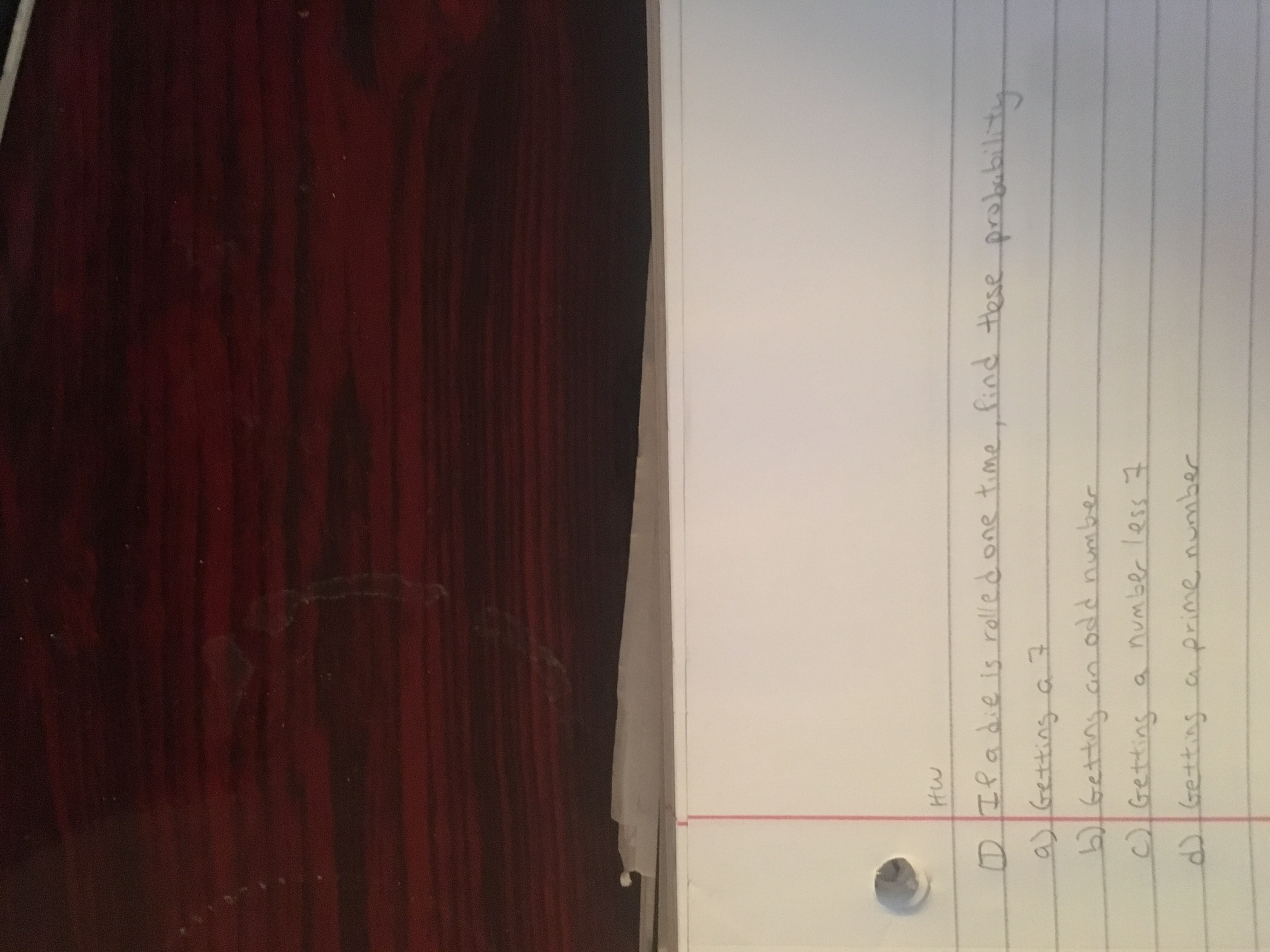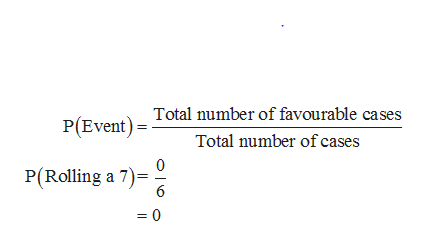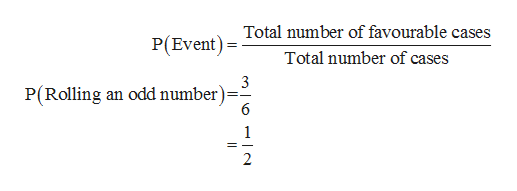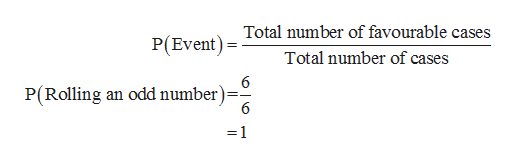# Hwa ০ 1 roe০০৫ +ne f +o2 rs ০।ఉలార్యు ఉవితట২০ ০ nnumber less 1GrettinsGetting ca prime number

Question
1 views

Can you please solve this question and show the stepshelp_outlineImage TranscriptioncloseHw a ০ 1 roe০০৫ +ne f +o2 r s ০। ఉలార్యు ఉవితట ২ ০ ০ n number less 1 Grettins Getting ca prime number fullscreen
check_circle

star
star
star
star
star
1 Rating
Step 1

a. Finding the probability of getting 7:

It is given that die is rolled one time.

When a six sided die is rolled one time, the probability of each outcome is 1/6.

The sample space when a die is rolled is {1,2,3,4,5,6} and all outcomes are equally likely.

The sample space does not contain the value 7. Thus, the probability of rolling a 7 is 0.

In other words, the probability that the die comes up value 7 is obtained as follows:help_outlineImage TranscriptioncloseP(Event) Total number of favourable cases Total number of cases P(Rolling a 7) 6 =0 fullscreen
Step 2

b. Finding the probability of getting an odd number:

The sample space contains three odd numbers. That is, 1, 3 and 5.

Thus, the probability that the die comes up value that is an odd number is obtained as follows:help_outlineImage TranscriptioncloseTotal number of favourable cases P(Event Total number of cases 3 P(Rolling an odd number) 6 1 2 fullscreen
Step 3

c. Finding the probability of getting a number less than 7:

The sample space contains 6 numbers that are less than 7. That is, 1, 2, 3, ...help_outlineImage TranscriptioncloseTotal number of favourable cases P(Event Total number of cases P(Rolling an odd number) =1 fullscreen

### Want to see the full answer?

See Solution

#### Want to see this answer and more?

Solutions are written by subject experts who are available 24/7. Questions are typically answered within 1 hour.*

See Solution
*Response times may vary by subject and question.
Tagged in

### Probability